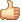# 雅乐网

## Andrew Ng机器学习课程笔记3——逻辑回归和正则化

### 逻辑回归（Logistic Regression）

#### 假说模型

$$h(x) = \theta^T x$$$$h(x) = g(\theta^T x)$$

$$g(z) = \frac{1}{1 + e^{-z}}$$$$h_\theta(x) = \frac{1}{1 + e^{- \theta^T x}}$$

$$h(x) = P(y=1 | x; \theta)$$

$$\theta^T x > 0$$时，对应h(x) > 0.5，应该预测结果为1；

$$\theta^T x < 0$$时，对应h(x) < 0.5，应该预测结果为0；

#### 决策边界（Decision Boundary）#### 代价函数（Cost Function）

$$J(\theta_0, \theta_1, …, \theta_n) = \frac{1}{2m} \sum_{i=0}^{m} (h(x^{(i)}) – y^{(i)})^2$$

$$J(\theta) = \frac{1}{m} \sum_{i = 1}^{m} \left( Cost(h(x^{(i)}), y^{(i)})\right)$$

$$Cost(h(x), y) = \begin{cases} -log(h(x)), & \text{if y = 1} \\ -log(1-h(x)), & \text{if y = 0} \\ \end{cases}$$

h(x)和Cost(h(x), y)之间的关系如下：$$Cost(h(x), y) = -y log(h(x)) – (1-y) log(1-h(x))$$

$$J(\theta) = – \frac{1}{m} \sum_{i = 1}^{m} \left( y^{(i)} log(h(x^{(i)})) + (1-y^{(i)}) log\left(1-h(x^{(i)})\right) \right)$$

$$\theta_j := \theta_j – \alpha \frac{\partial}{\partial \theta_j} J(\theta)$$

$$\theta_j := \theta_j – \frac{\alpha}{m} \sum_{i=1}^{m}\left( \left(h(x^{(i)}) – y^{(i)}\right) x_j^{(i)} \right)$$

$$h(x) = \theta^T x$$

$$h(x) = g(\theta^T x) = \frac{1}{1 + e^{- \theta^T x}}$$

#### 调用matlab中的高级算法#### 多分类问题$$h^{(i)}(x)$$

### 正则化（Regularization）

#### 过拟合（Overfitting）#### 正则化代价函数

$$h(x) = \theta_0 + \theta_1 x1 + \theta_2 x_2^2 + \theta_3 x_3^3 + \theta_4 x_4^4$$

$$J(\theta) = \frac{1}{2m} \left( \sum_{i=1}^{m} (h(x^{(i)}) – y^{(i)})^2 + 999\theta_3^2 + 999\theta_4^2 \right)$$

$$J(\theta) = \frac{1}{2m} \left( \sum_{i=1}^{m} (h(x^{(i)}) – y^{(i)})^2 + \lambda \sum_{j=1}^{n} \theta_j^2 \right)$$

#### 正则化线性回归

$$J(\theta) = \frac{1}{2m} \left( \sum_{i=1}^{m} (h(x^{(i)}) – y^{(i)})^2 + \lambda \sum_{j=1}^{n} \theta_j^2 \right)$$

$$\theta_0 := \theta_0 – \alpha \frac{1}{m} \sum_{i=1}^{m} \left( (h(x^{(i)}) – y^{(i)}) x_0^{(i)} \right)$$

$$\theta_j := \theta_j – \alpha \left( \frac{1}{m} \sum_{i=1}^{m} (h(x^{(i)}) – y^{(i)}) x_0^{(i)} + \frac{\lambda}{m} \theta_j \right) , \text{for j = 1, 2, … , n}$$

$$\theta_j := \theta_j(1 – \alpha \frac{\lambda}{m}) – \alpha \frac{1}{m} \sum_{i=1}^{m} \left( (h(x^{(i)}) – y^{(i)}) x_j^{(i)} \right)$$#### 正则化逻辑回归

$$J(\theta) = – \left[ \frac{1}{m} \sum_{i = 1}^{m} \left( y^{(i)} log(h(x^{(i)})) + (1-y^{(i)}) log\left(1-h(x^{(i)})\right) \right) \right] + \frac{\lambda}{2m} \sum_{j=1}^{n} \theta_j^2$$

$$\theta_0 := \theta_0 – \alpha \frac{1}{m} \sum_{i=1}^{m} \left( (h(x^{(i)}) – y^{(i)}) x_0^{(i)} \right)$$

$$\theta_j := \theta_j – \alpha \left(\frac{1}{m} \sum_{i=1}^{m} (h(x^{(i)}) – y^{(i)}) x_j^{(i)} + \frac{\lambda}{m} \theta_j \right) , \text{for j = 1, 2, … , n}$$

https://www.yalewoo.com/andrew_ng_machine_learning_notes_3_logistic_regression_and_regularization.html

### 文章《Andrew Ng机器学习课程笔记3——逻辑回归和正则化》共有2条评论：

1.dgs

不是归一化线性/逻辑回归, 是正则化线性/逻辑回归. 归一化是mean normalization, 正则化是regularization. 两个不是一回事

•yalewoo 作者

对的对的 谢谢指正已经修改了